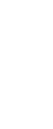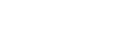# KASSU JOINT EVALUATION EXAMINATION CHEMISTRY Paper 1 with answers Featured

1. Give two differences between luminous and non-luminous flames. (2 marks)

 Luminous Non-luminous - Bright yellow flame- Sooty- 4-regions- Large and wavy - Blue flame- Non-sooty- 3-region- Short and steady

2. How is the non-luminous flame produced? (1 mark)
- When the air hole is fully open.

1. The apparatus below were used to separate a mixture of liquid A and B.1. State two properties of liquids that make it possible to separate using such apparatus. (2 marks)
- Immiscible
- Different densities

2. Give the name of the above apparatus. (1 mark)
- Separating funnel

1. Explain why solid Carbon (IV) oxide is preferred over ordinary ice for use by ice cream venders. (1 mark)
- Better coolant
- Sublims leaving no wetness

2. Name one piece apparatus used to measure volume of gases. (1 mark)
- Syringe

3. Draw a diagram of a deflagrating spoon. (1 mark)2. The table below shows the pH values of solutions P, R, Q and S.

 Solution P R Q S pH 2 7 6.5 13.5

1. Which solution represent:
(i) Strong base - S (1 mark)
(ii) Weak acid - Q (1 mark)

2. Give an example of solution S. (1 mark)
- Sodium hydroxide/potassium hydroxide

3. 6.95g of hydrated iron (II) sulphate FeSO4. nH2O was dissolved in 250 cm3 solution resulting into a 0.1M solution. Determine the value of n. (3 marks) (Fe = 56, O = 16, S = 32, H = 1).

Moles of soln
If 0.1 moles are in 1000cm34. Rusting leads to fast wearing out of farm tools and equipment as well as buildings.
1. Give the chemical name of rust. (1 mark)
- Hydrated Iron (III) oxide

2. What two conditions accelerate rusting process? (2 marks)
- High temperatures
- Acidic conditions
- Salty conditions

5. Study the diagram below and answer the questions that follow.1. Write an equation for the reaction that take place in the combustion tube. (1 mark)
- 3Fe(s) + 4H2O(g)------> Fe3O4(s) + 4H2(g)

2. Why would it not be advisable to use potassium in place of iron in the set-up? (1 mark)
- It is more reactive, hence reacts explosively with steam.

3. Glass wool should be heated before heating iron. Explain. (1 mark)
- So as to generate/evolve steam first

1. Name the following organic compounds.
1.2-bromo-4-methyl pentane

2. HOCH2 - CHOH - CH2OH (1 mark)
Propen-1, 2, 3 - trio1

2. Given
1. CH3(CH2)16 COO- Na+
2. CH3(CH2)6 CH(CH3)CH2 SO-3 Na+
Identify detergent
a - Soapy (1 mark)
b - Soapless (1 mark)

6. In terms of structure and bonding, explain the following.
1. Graphite is used as a lubricant. (1 mark)
- The hexagonal layers are joined by weak van der wals forces which can slide over each other easily is the giant cordent structure.

2. Alluminium is better conductor of electricity then magnesium. (1 mark)
- Has more delocalized electrons in their giant metallic structure.

3. Water is a liquid at room temperature while hydrogen sulphide is a gas. (1 mark)
- Water has strong hydrogen bonds as compared to the weak van der wals forces in H2S in their molecular structure.

1. Define the term molar latent heat of fusion. (1 mark)
- Molar latent heat of fusion of a substance is the amount of heat absorbed when one mole of the solid is converted into the liquid state at constant temperature and pressure.

2. The molar heat of fusion of ice at O0C is 6kJ mol-1. Calculate the heat change when 36g of ice is converted to 36g of water at 100C. (3 marks) (SHC = 4.2-1g K-1, density = 1.0g/cm3, H = 1.0, O = 16.0)7. Draw a well labeled diagram showing how blister copper is purified. (3 marks)8. Gas Q with a relative molecular mass of 48 took 50 seconds to diffuse through a porous diaphragm. How long will it take for the same amount of hydrogen Chloride (HCl) to diffuse through the same diaphragm under similar conditions? (H = 1.0, Cl = 35.5). (3 marks)1. Calculate the oxidation state of chromium in the ion Cr2 O2-. (1 mark)
2cr + -2 x 7 = -2
2cr - 14 = -2
2cr = +12
Cr = +6

2. Using oxidation numbers, determine from the equation below the species which undergoes oxidation and reduction.
2FeCl2(aq) + Cl2(g) 2Fe Cl3(aq)
Oxidation - Fe2+ (Iron (II) ions) (1 mark)
Reduction - Chlorine (1 mark)

9. Given elements A, B and C with atomic numbers 11, 19 and 13 respectively.
1. Compare the atomic radius of A and C. Explain. (2 marks)
- C is smaller than A. It has more protons which increases nuclear attraction.

2. Compare reactivity of A and B. (1 mark)
- B is more reactive than A.

10. Haber process (the manufacture of ammonia gas) is given by the following equation.
N2(g) + 3H2(g) <======> 2NH3(g)    ΔH = -92kJ mol-1.
State and explain the effect of:
1. Introducing some drops of water to the equilibrium. (1 mark)
- The equilibrium shifts to the right to replace the ammonia gas absorbed by water molecules.

2. Pumping nitrogen gas to the equilibrium mixture. (1 mark)
- The equilibrium shifts to the right to use up the nitrogen gas added.

3. Lowering the temperature of the reaction. (1 mark)
- Forward reaction if exothermic hence favoured by low temperatures. Therefore the equilibrium shifts to the right.

11. Elements P and Q have the following atomic numbers 19 and 8 respectively.
(i) Using dot (.) and cross(x) draw a diagram to show how the elements form bonds. (1 mark)12. Describe how sodium sulphate crystals can be prepared starting with 50cm3 of 2M sodium hydroxide and 1M sulphuric (VI) acid. (3 marks)
- Moles of NaOH = 0.1 moles
2NaOH(aq) + H2SO4(aq) --------> Na2SO4(aq) + 2 H2O(l)
Moles of H2SO4 required = 0.05 moles
Volume of H2SO4 required = 0.05 = 50cm3
- Measure 50cm3 of 1M H2SO4 and add it to 50cm3 of 2M NaOH(aq) in a beaker.
- Heat the mixture to concentrate the solution.
- Allow the concentrated solution to evaporate slowly to form crystals of sodium sulphate.

13. Write ionic equations to show how;
1. (i) Excess ammonia solution reacts with a solution containing Copper II ions. (1 mark)
Cu(OH)2(s) + 4NH3(aq) ---------->[Cu(NH3)4]2+(aq) + 2OH-(aq)
(ii) Excess sodium hydroxide added to a solution containing Al3+ ions.
Al(OH)3(s) + OH-(aq)-------------> [Al(OH)4]-(aq)

2. Give the name of the following ion [Zn(NH3)4]2+ (1 mark)
- Tetramine zinc ions.

1. Define electrolysis. (1 mark)
- Process of the decomposition of an electrolyte by passing electric current through it.

2. During the electrolysis of molten aluminium oxide, write the equations at the;
Anode - 6O2-(l) 3O2(g) + 12e- (1 mark)
Cathode - 4Al3+ + 12e- 4Al(s). (1 mark)

1. Give any two differences between alpha and beta particles. (2 marks)

 Alpha Beta Positively charged Very Charged Heavy Light

2. A radioactive isotope T decays by emitting three alpha particles to form what is the atomic number and mass number T?
Atomic number - 89 (1 mark)
Mass number - 226 (1 mark)
1. Using acidified potassium dichromate (VI) solution, describe how you would differentiate between sulphur (IV) oxide and hydrogen sulphide. (2 marks)
- Bubble the gases into separate test tube containing acidified potassium dichromate (VI) solution; Both change potassium dichromate (VI) solution from orange to green, but a yellow solid overdue/deposited is formed with hydrogen sulphide no yellow deposit formed with sulphur (IV) oxide.

2. Identify the catalyst preffered in ......... Explain (2 marks)
- Platinum and vanadium (V) oxide.
- Vanadium (V) oxide is preferred because it is cheaper and less easily poisoned.
Ammoniated brine

14. Study the following part of the solvay process for the manufacture of sodium carbonate and answer the questions that follows:(i) State the main source of Carbon (IV) oxide in the process. (1 mark)
- (Heating) coke/calcium carbonate.
(ii) Write down the overall equation for the reaction in chamber I. (1 mark)
NaCl(aq) + NH3(g) + H2O(l) + CO2(g) -----------------> NaHCO3(s) + NH4Cl(aq)
(iii) Name process in step 1. (1 mark)
- Cooling
- The reaction is exothermic

15. The following equation involve hydrochloric acid.
MnO2(s) + 4HCl(aq)-----------------> MnCl2(aq) + 2H2O(l) + Cl2(g)
1. State the type of reaction taking place in the reaction. (1 mark)
...............................................

2. State two contrasting chemical properties of hydrogen and chlorine. (2 marks)

 Hydrogen Chlorine Reducing agent Oxidising agent Does not bleach Bleaching agent
1. An element O has 2 isotopes containing 90% and Isotope .
(i) What are isotopes? (1 mark)
- They are atoms of some elements with some atomic number but different mass number due to different number of neutrons.
(ii) Find the R.A.M of O. (2 marks)16. When a hydrocarbon is completely burnt in oxygen 4.2g of Carbon (IV) oxide and 1.71g of water were formed.
1. Determine the empirical formular of the hydrocarbon. (3 marks)2. Given that formula mass of compound above is 28. Find the molecular formular. (1 mark)
(CH2)n = 28
14n = 28
n = 2
C2H4

1. Name the two types of polymerization. (1 mark)
- Substitution

2. Study the section of the polymer below and answer the questions that follow.(i) Give the name of the polymer above. (1 mark)
- Polyphenylethene/ polyslyrene

• ✔ To read offline at any time.
• ✔ To Print at your convenience
• ✔ Share Easily with Friends / Students